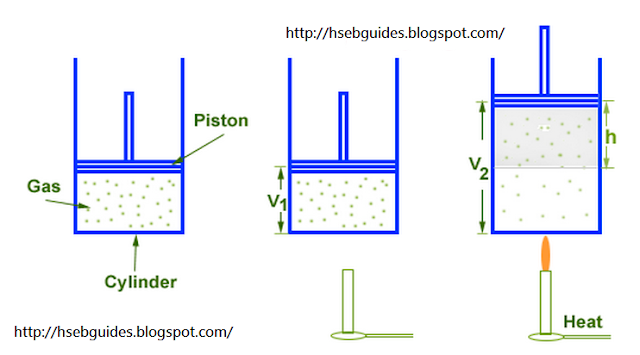# Pressure-Volume Work

Consider a cylinder fitted with a frictionless and weightless non-conducting piston of area of cross section “A“. An ideal gas is enclosed in the cylinder. Let the volume of gas at initial state is “V1“. An external pressure "P" is exerted on the piston.If we supply “q” amount of heat to the system then it will increase its internal energy by DE. “After a certain limit gas exerts pressure on the piston. If piston is free to move, it will be displaced by “h” & volume of system increases from V1 to V2.

We know that pressure is the force per unit area i.e.
P = F/A
OR
F = PA …….. (i)
We also know that the work done by the gas on the piston is given by:
DW = F d

Where d = displacement of piston = h
Putting the value of F and d ,we get
DW = (PA) h
OR DW = P (Ah)
But Ah = change or increase in volume = DV
Hence
DW = P DV